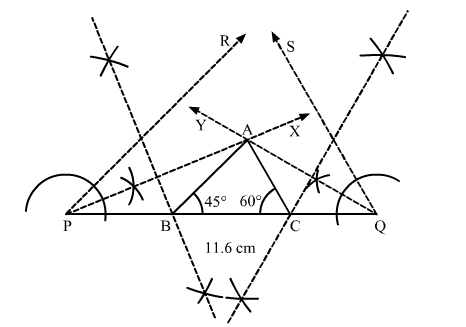# Construct a ∆ABC whose perimeter is 11.6 cm and the base angles are 45° and 60°

Question:

Construct a ∆ABC whose perimeter is 11.6 cm and the base angles are 45° and 60°

Solution:Steps of construction:
1. Draw a line segment PQ = 11.6 cm.

2. Construct an angle of $45^{\circ}$ and bisect it to get $\angle Q P X$.

3. Construct an angle of $60^{\circ}$ and bisect it to get $\angle P Q Y$.

4. The ray XP and YQ intersect at A.
5. Draw the right bisectors of AP and AQ, cutting PQ at B and C, respectively.
6. Join AB and AC.

Thus, $\triangle A B C$ is the required triangle.